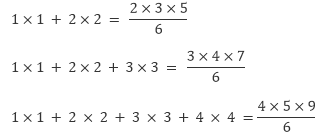×#### Thank you for registering.

One of our academic counsellors will contact you within 1 working day.

Click to Chat

1800-1023-196

+91-120-4616500

CART 0

• 0

MY CART (5)

Use Coupon: CART20 and get 20% off on all online Study Material

ITEM
DETAILS
MRP
DISCOUNT
FINAL PRICE
Total Price: Rs.

There are no items in this cart.
Continue Shopping• Complete JEE Main/Advanced Course and Test Series
• OFFERED PRICE: Rs. 15,900
• View Details

```Chapter 4: Operations on Whole Numbers – Exercise 4.5

Question: 1

Without drawing a diagram, find:

Solution:

(i) 10th square number:

A square number can easily be remembered by the following rule

Nth square number = n × n

10th square number = 10 × 10 = 100

(ii) 6th triangular number:

A triangular number can easily be remembered by the following rule

Nth triangular number = n × ( n + 1 )2

Therefore, 6th triangular number = 6 × (6 + 1)2 = 21

Question: 2

(i) Can a rectangle number also be a square number?

(ii) Can a triangular number also be a square number?

Solution:

(i) Yes, a rectangular number can also be a square number; for example, 16 is a square number also a rectangular number.(ii) Yes, there e×ists only one triangular number that is both a triangular number and a square number, and that number is 1.

Question: 3

Write the first four products of two numbers with difference 4 starting from in the following order:

1 , 2 , 3 , 4 , 5 , 6 , ………..

Identify the pattern in the products and write the next three products.

Solution:

1 × 5 = 5 (5 – 1 = 4)

2 × 6 = 12 (6 – 2 =4)

3 × 7 = 21 (7 – 3 = 4)

4 × 8 = 32 (8 – 4 = 4)

Question: 4

Observe the pattern in the following and fill in the blanks:

Solution:

9 × 9 + 7 =88

98 × 9 + 6 = 888

987 × 9 + 5 = 8888

9876 × 9 + 4 = 88888

98765 × 9 + 3 = 888888

987654 × 9 + 2 = 8888888

9876543 × 9 + 1 = 88888888

Question: 5

Observe the following pattern and extend it to three more steps:

Solution:

6 × 2 – 5 = 7

7 × 3 – 12 = 9

8 × 4 – 21 = 11

9 × 5 – 32 = 13

10 × 6 – 45 = 15

11 × 7 – 60 = 17

12 × 8 – 77 = 19

Question: 6

Study the following pattern:

1 + 3 = 2 × 2

1 + 3 + 5 = 3 × 3

1 + 3 + 5 + 7 = 4 × 4

1 + 3 + 5 + 7 + 9 = 5 × 5

By observing the above pattern, find:

Solution:

(i) 1 + 3 + 5 + 7 + 9 + 11

= 6 × 6

= 36

(ii) 1 + 3 + 5 + 7 + 9 + 11 + 13 + 15

= 8 × 8

= 64

(iii) 21 + 23 + 25 + … + 51

= (21 + 23 + 25 +…+ 51) can also be written as

(1 + 3 + 5 + 7 +…+ 49 + 51) – (1 + 3 + 5 +…+ 17 + 19)

(1 + 3 + 5 + 7+…+ 49 + 51) = 26 × 26 = 676

and, (1 + 3 + 5 +…+ 17 + 19 ) = 10 × 10 = 100

Now,

(21 + 23 + 25 +…+ 51 ) =  676  – 100 = 576

Question: 7

Study the following pattern:By observing the above pattern, write next two steps.

Solution:

The next two steps are as follows:

1 × 1 + 2 × 2 + 3 × 3 + 4 × 4 + 5 × 5

= 5 × 6 × 116

= 55

1 × 1+ 2 × 2 + 3 × 3 + 4 × 4 + 5 × 5 + 6 × 6

= 6 × 7 × 136

= 91

Question: 8

Study the following pattern:By observing the above pattern, find:

Solution:

(i) 1 + 2 + 3 + 4 + 5 + 6 + 7 + 8 + 9 + 10

= 10 × 112

= 55

(ii) 50 + 51 + 52 + …+ 100

This can also be written as

(1 + 2 + 3 + …+ 99 + 100) – (1 + 2 + 3 + 4 + …+ 47 + 49)

Now,

(1 + 2 + 3 + …+ 99 + 100 ) = 100 × 1012

and, (1 + 2 + 3 + 4 +…+ 47 + 49 ) = 49 × 502

So, (50 + 51 + 52 + …+ 100 ) = 100 × 1012 – 49 × 502

= 5050 – 1225

= 3825

(iii) 2 + 4 + 6 + 8 + 10 +…+ 100

This can also be written as 2 × (1 + 2 + 3 + 4 + …+ 49 + 50)

Now,

(1 + 2 + 3 + 4 + …+ 49 + 50 ) = 50 × 512

= 1275

Therefore, (2 + 4 + 6 + 8 + 10 + …+ 100) = 2 × 1275 = 2550
```### Course Features

• 728 Video Lectures
• Revision Notes
• Previous Year Papers
• Mind Map
• Study Planner
• NCERT Solutions
• Discussion Forum
• Test paper with Video Solution